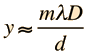# Double Slit InterferenceMultiple slits Include diffraction Examine approximation Calculation Single slit diffraction Comparison to single slit
Index

Interference concepts

 HyperPhysics***** Light and Vision R Nave
Go Back

# Double Slit InterferenceDisplacement y = (Order m x Wavelength x Distance D)/(slit separation d)

For double slit separation d = micrometers = x10^ m

 and light wavelength λ = nm at order m =,

on a screen at distance D = cm

the displacement from the centerline for maximum intensity will be= cm.

 This corresponds to an angle of θ = ° .

This calculation is designed to allow you to enter data and then click on the quantity you wish to calculate in the active formula above. The data will not be forced to be consistent until you click on a quantity to calculate. Default values will be entered for unspecified parameters, but all values may be changed.

Note: The small angle approximation was not used in the calculations above, but it is usually sufficiently accurate for laboratory calculations.

 Multiple slits Include diffraction Examine approximation
Index

Interference concepts

 HyperPhysics***** Light and Vision R Nave
Go Back